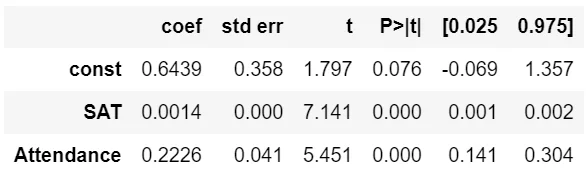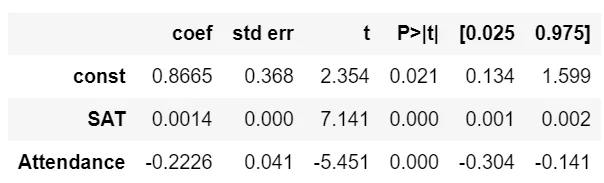02 Nov 2021

Posted on:

02 Nov 2021

0

# Should we consider the numerical value aligned to categorical datas?

I have one question related to the above example. We are all aware that students with higher attendance usually have higher GPA. The presenter has reasonably mapped the Attended to 1 and the Didn't attend to 0 (1>0), which just makes sense and we receive two perfect paralleled lines representing the two categories. However, what if we reverse the case? For instance, we mapped Attended as 0 and Unattended as 1 or even change the case to Attended as -1 and Unattended to +1. What would be the results then? Will we still get the lines paralleled? Will there be any significant changes in the coefficient of other variables (Const and SAT Score)? And to what extent do our model better off fit the data set or does it becomes less reliable? All in all, I do understand the concept of Dummy Variables, however, should we closely consider the numerical values that we will align our categorical data with?

Instructor
Posted on:

02 Nov 2021

0

Hey,

The choice of where we put the 0 and where we put the 1 is completely arbitrary. The only difference would be in the coefficients in the end.

Here are the results for the mapping Yes:1, No:0:Therefore, the two equations will be:

GPA_attends = 0.6439 + 0.0014 * SAT + 0.2226 * 1 = 0.8665 + 0.0014 * SAT
GPA_does_not_attend = 0.6439 + 0.0014 * SAT + 0.2226 * 0 = 0.6439 + 0.0014 * SAT

As you've seen, this results in two parallel lines, the higher one corresponding to the function GPA_attends(SAT).

Now, let's map the variables as follows - Yes:0, No:1:Therefore, the two equations will now be:
GPA_attends = 0.8665 + 0.0014 * SAT + 0.2226 * 0 = 0.8665 + 0.0014 * SAT
GPA_does_not_attend = 0.8665 + 0.0014 * SAT - 0.2226 * 1 = 0.6439 + 0.0014 * SAT

This would result in the same parallel lines once the functions are plotted.

Hope this helps!

Kind regards,
365 Hristina